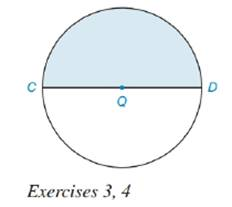Chapter 8.5, Problem 3EElementary Geometry For College St...

7th Edition
Alexander + 2 others
ISBN: 9781337614085

Solutions

Chapter
SectionElementary Geometry For College St...

7th Edition
Alexander + 2 others
ISBN: 9781337614085
Textbook Problem

In the semicircular region shaded D Q = 6 " .a) Find the exact perimeter of the region.b) Find the exact area of the region.To determine

(a)

To find:

The exact perimeter of the shaded region.

Explanation

Definition:

Semicircle is one half of the circle.

Since the semicircular region corresponds to a central angle of 180, the perimeter of semicircle is one half of the circumference of the circle.

Formula:

If r is the radius of circle,

Psemicircle=12× Circumference of circle =12×2πr=πr.

Thus, Psemicircle=πr.

Calculation:

Given that DQ=6. Thus, radius of circle measures 6.

Let’s substitute the value of r as 6 in the formula

To determine

(b)

To find:

The exact area of the shaded region.

Still sussing out bartleby?

Check out a sample textbook solution.

See a sample solution

The Solution to Your Study Problems

Bartleby provides explanations to thousands of textbook problems written by our experts, many with advanced degrees!

Get Started

In Exercises 4562, find the values of x that satisfy the inequality (inequalities). 50. 0 x + 2 4

Applied Calculus for the Managerial, Life, and Social Sciences: A Brief Approach

Convert from radians to degrees. 12. 5

Single Variable Calculus: Early Transcendentals, Volume I

Subtract and check: 417,286287,156

Elementary Technical Mathematics

f(x) = 12x x3 has a local maximum at: a) x = 0 b) x = 2 c) x = 2 d) f does not have a local maximum

Study Guide for Stewart's Single Variable Calculus: Early Transcendentals, 8th